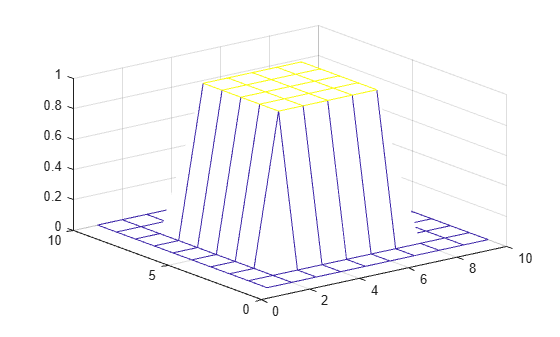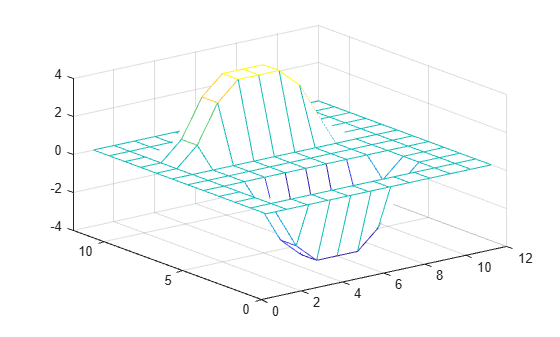# filter2

## 语法

``Y = filter2(H,X)``
``Y = filter2(H,X,shape)``

## 说明

``Y = filter2(H,X)` 根据矩阵 `H` 中的系数，对数据矩阵 `X` 应用有限脉冲响应滤波器。`

``Y = filter2(H,X,shape)` 根据 `shape` 返回滤波数据的子区。例如，`Y = filter2(H,X,'valid')` 仅返回计算的没有补零边缘的滤波数据。`

## 示例

```A = zeros(10); A(3:7,3:7) = ones(5); mesh(A)``````H = [1 2 1; 0 0 0; -1 -2 -1]; Y = filter2(H,A,'full'); mesh(Y)````H` 旋转 180 度，并将结果与 `A` 进行卷积。该输出等同于按照 `H` 中的系数对 `A` 中的数据进行滤波。

```C = conv2(A,rot90(H,2)); mesh(C)```## 输入参数

• `'same'` - 返回滤波数据的中心部分，大小与 `X` 相同。

• `'full'` - 返回完整的二维滤波数据。

• `'valid'` - 仅返回计算的没有补零边缘的滤波数据部分。

## 算法

`filter2` 函数通过取输入 `X` 的二维卷积和旋转 180 度的系数矩阵 `H` 对数据进行滤波。具体而言，就是 `filter2(H,X,shape)` 等同于 `conv2(X,rot90(H,2),shape)`